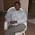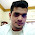Skip to main content

### AX 2012 / AX 2009: Layers object

Hi folks J today am going to illustrate the sample code which helps you in finding all the different objects in the specified layers.

So Running the below code opens a dialog wherein we can select the layer as shown:

AX2012:

static void LayerObjectsAX2012(Args _args)
{
#AOT
treeNode treeNode;
xInfo xInfo = new xInfo();
UtilElements utilElements;
str layer;
int counter = 0;
textBuffer textBuffer = new textBuffer();
dialog dialog = new dialog("Find the modified objects");
dialogfield layerTypeDf;
;
layerTypeDf = dialog.addField(enumStr(utilEntrylevel),"Choose the layer");
if(dialog.run())
{
treeNode = treenode::findNode(#TablesPath);

treeNode = treeNode.AOTfirstChild();

while (treeNode)
{
if(SysTreeNode::existsInLayer(treeNode, layerTypeDf.value()))
{

textBuffer.appendText(treeNode.AOTname());
textBuffer.appendText('\n');
counter ++;
}
treeNode = treeNode.AOTnextSibling();

}
}
if(strlen(textbuffer.getText()) > 0)
{
textBuffer.toFile(@"C:\Users\vtiwari\Desktop\TablemodifiedObjectInUSR.txt");
info("Total modified objects in usr layer are " + int2str(counter));
}
}

On running the above script, creates a text file on the specified location which comprise of all the selected layers object.

Below script does the similar function in AX2009 as described above.
AX2009:

static void LayerObjectsAX2009(Args _args)
{
treeNode treeNode;
xInfo xInfo = new xInfo();
UtilElements utilElements;
str layer;
int counter = 0;
textBuffer textBuffer = new textBuffer();
dialog dialog = new dialog("Find the modified objects");
dialogfield layerTypeDf;
;
layerTypeDf = dialog.addField(Typeid(utilEntrylevel),"Please choose the layer");
if(dialog.run())
{
treeNode = treenode::findNode('\\Macros');
if(treeNode.AOTIsOld())
{

}
treeNode = treeNode.AOTfirstChild();

while (treeNode)
{
if(treeNode.applObjectLayer()==  layerTypeDf.value())
{

textBuffer.appendText(treeNode.AOTname());
textBuffer.appendText('\n');
counter ++;
}
treeNode = treeNode.AOTnextSibling();

}
}
if(strlen(textbuffer.getText()) > 0)
{
textBuffer.toFile("c:\\modifedObjectsUSRLayer.xls");
info("Total modified objects in usr layer are " + int2str(counter));
}

}

Happy Dax6ng J

### Comments

1.Hey Tiwari.....Good blog.

1.Thanks Raj :)

2.Alternative way is to create new project in AOT. Press filter button. Select layer in query. Press OK.
AX will fill this project according to your query.
This is standard way in AX to find modified objects.